## RD Sharma Class 8 Solutions Chapter 14 Compound Interest Ex 14.5

These Solutions are part of RD Sharma Class 8 Solutions. Here we have given RD Sharma Class 8 Solutions Chapter 14 Compound Interest Ex 14.5

Other Exercises

Question 1.
Mr. Cherian purchased a boat for Rs 16,000. If the total cost of the boat is depreciating at the rate of 5% per annum, calculate its value after 2 years.
Solution:
Cost of boat = Rs 16,000
Rate of depreciating = 5% p.a.
Period = 2 years
Value of boat after 2 years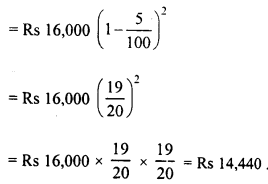Question 2.
The value of a machine depreciates at the rate of 10% per annum. What will be its value 2 years hence, if the present value is Rs 1,0,000 ? Also, find the total depreciation during this period.
Solution:
Present value of machine = Rs 1,00,000
Rate of depreciation = 10% p.a.
Period (n) = 2 years
Value of machine after 2 years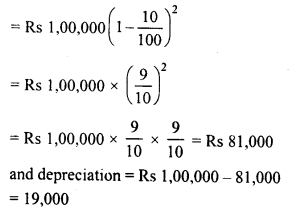Question 3.
Pritam bought a plot of land for Rs 6,40,000. Its value is increasing by 5% of its previous value after every six months. What will be the value of the plot after 2 years ?
Solution:
Present value of plot = Rs 6,40,000
Increase = 5% per half year
Period (n) = 2 years or 4 half years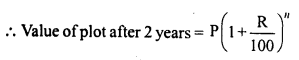Question 4.
Mohan purchased a house for Rs 30,000 and its value is depreciating at the rate of 25% per year. Find the value of the house after 3 years.
Solution:
Present value of the house (P) = Rs 30,000
Rate of depreciation = 25% p.a.
Period (n) = 3 years
Value of house after 3 yearsQuestion 5.
The value of a machine depreciates at the rate of 10% per annum. It was purchased 3 years ago. If its present value is Rs 43,740, find its purchased price.
Solution:
Let the purchase price of machine = Rs P
Rate of depreciation = 10% p.a.
Period (n) = 3 years.
and present value = Rs 43,740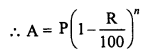Question 6.
The value of a refrigerator which was purchased 2 years ago, depreciates at 12% per annum. If its present value is Rs 9,680, for how much was it purchased ?
Solution:
Let the refrigerator was purchased for = Rs P
Rate of depreciation (R) = 12% p.a.
Period (n) = 2 years
and present value (A) = Rs 9,680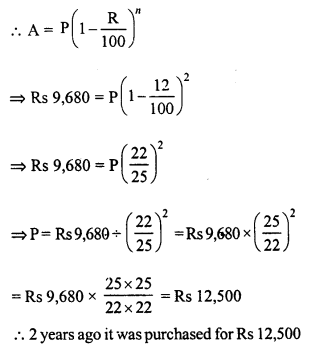Question 7.
The cost of a TV set was quoted Rs 17,000 at the beginning of 1999. In the beginning of2000, the price was hiked by 5%. Because of decrease in demand the cost was reduced by 4% in the beginning of 2001. What is the cost of the TV set in 2001 ?
Solution:
List price of TV set in 1999 = Rs 17,000
Rate of hike in 2000 = 5%
Rate of decrease in 2001 = 4%
Price of TV set in 2001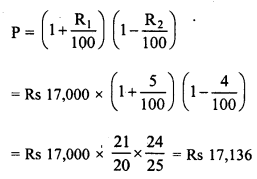Question 8.
Ashish started the business with an initial investment of Rs 5,00,000. In the first year, he incurred a loss of 4%. However, during the second year he earned a profit of 5% which in third year, rose to 10%. Calculate the net profit for the entire period of 3 years.
Solution:
Initial investment = Rs 5,00,000
In the first year, rate of loss = 4%
In the second year, rate of gain = 5%
and in the third year, rate of gain = 10%
Investment after 3 yearsHope given RD Sharma Class 8 Solutions Chapter 14 Compound Interest Ex 14.5 are helpful to complete your math homework.

If you have any doubts, please comment below. Learn Insta try to provide online math tutoring for you.